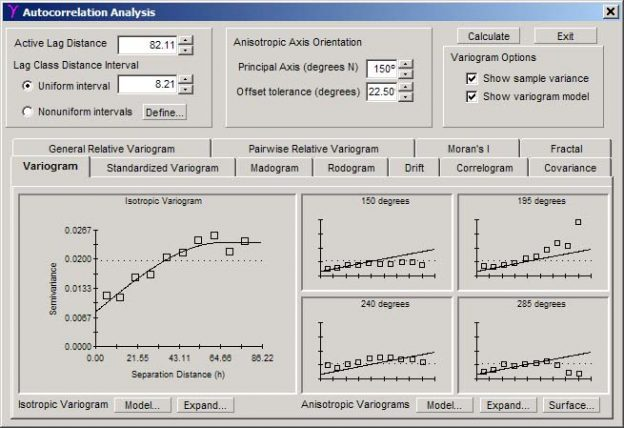# GS+ Semivariance Analysis (Variography)

GS+ Variograms
Variograms produced by GS+ appear initially in the Autocorrelation Window. By pressing the Enlarge command in that window, a full-window variogram like the one below is created. From this window the variogram can be edited and printed. Additionally, the semivariance values that were used to produce the variogram can be listed, and Variance Cloud and h-Scattergram analyses provide a way to detect outlier pairs of points that may be artificially skewing the variogram (such as the point under the cursor at the 7th lag class, to the right).

Different anisotropic directions appear on separate tabs. Parameters for calculating variograms are set in the Autocorrelation Window, where it is also possible to access the Model Definition window to adjust the modeled line drawn amongst variogram points.

When the program is running, placing the mouse cursor over a variogram point will produce a box showing the number of pairs in that lag class interval, and clicking on the point will produce a Variance Cloud or h-Scattergram graph of all pairs in that interval.

Other measures of autocorrelation provided by GS+ include correlograms, madograms, rodograms, covariograms, drift, Moran’s I, fractal dimension, and standardized, general relative, and pairwise relative variograms.

Anisotropic Variogram Map (2D) in GS+
The Anisotropic Variogram Surface or Variogram Map provides a visual picture of semivariance in every compass direction. This allows one to more easily find the appropriate principal axis for defining the anisotropic variogram model. A transect in any single direction (e.g. 10 degrees north) is equivalent to the variogram in that direction: the surface (z-axis) is semivariance; the x and y axes are separation distances in E-W and N-S directions, respectively. The center of the map corresponds to the origin of the variogram for every direction. The map can also be projected as a 3d surface via the Edit Graph command. This window is accessed from the main Autocorrelation Analysis window.

GS+ Variance Cloud Analysis
A variance cloud is a graph of the variances associated with individual pairs of points in an autocorrelation analysis. It is most useful for discovering outliers that can skew the average semivariance value for a lag class. By placing the mouse on top of individual points you can determine which pairs of points in the data set are suspect.

Variance clouds are specific to both direction (isotropic or a specific anisotropic direction) and to a particular lag class. In the example below the cloud is specific to the 7th lag class of the isotropic variogram, as noted in the graph title, and the mouse is on top of the pair made up of data records 4 and 96, which are separated by 52.77 distance units. The List Values command lists numeric values for the cloud pairs in a separate Variance Cloud Listing window. When the program is running you can click on a graphed point and be given the option of removing one of the records from the analysis, as explained below.

Variance Cloud analysis is initiated by clicking on a semivariance point on a variogram in either the Autocorrelation Analysis window or the Variogram window.

GS+ h-Scattergram Analysis
In an h-Scattergram the individual values that make up a semivariance pair (the head value and the tail value) are plotted against each other for all the points that make up a variogram lag class. Like variance cloud graphs, h-Scattergrams are very useful for discovering outliers that can skew the average semivariance value for a particular lag class. By placing the mouse on top of individual points you can determine which pairs of points in the data set are suspect.

h-Scattergrams are specific to both direction (isotropic or a specific anisotropic direction) and to a particular lag class. In the example below the scattergram is specific to the 7th lag class of the isotropic variogram, as noted in the graph title, and the mouse is on top of a pair made up of data records 4 and 92, which are separated by 52.28 distance units. If you were to put the mouse over nearby points, you would learn that all the other points have record 4 in common. This suggests that record 4 is an outlier and might be excluded from subsequent analysis (as described below).

h-Scattergrams are created by clicking on a point in a variogram or autocorrelogram in either the Autocorrelation Analysis window or the Variogram window. The List Values command lists numeric values for the cloud pairs in a separate h-Scattergram Listing window.

GS+ Variance Cloud Listing
The Variance Cloud Listing provides a listing of all pairs of data points making up an average semivariance value (an individual point on the variogram). These are the pairs that are graphed in the Variance Cloud Window.

The listing provides for each pair of points within a specific lag class interval the variance, separation distance, and the identity (data record number) of each member of the pair. Individual pairs are graphed.